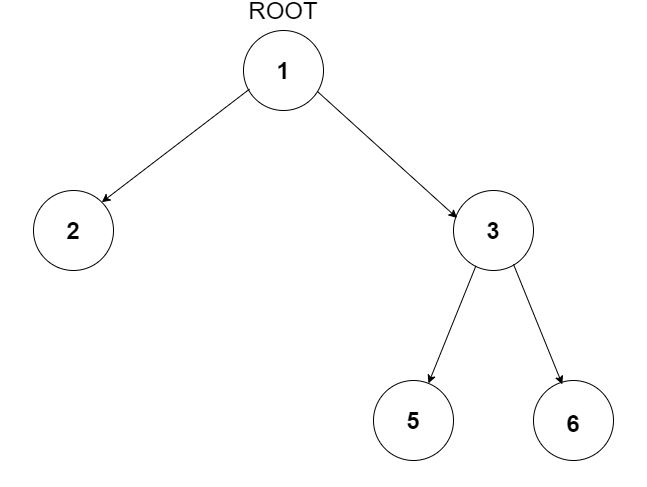Problem title
Difficulty
Avg time to solve

Path Reversals
Moderate
25 mins
Zigzag Binary Tree Traversal
Easy
10 mins
Vertical Order Traversal
Moderate
35 mins
Boredom
Moderate
25 mins
Smallest Window
Moderate
10 mins
Paths in a Maze
Easy
--
Positive Negative Pair
Easy
10 mins
Christmas Eve
Easy
15 mins
Left Rotations of An Array
Moderate
10 mins
Minimum Time To Solve The Problems
Hard
50 mins6

# Triplets in Binary Tree

Difficulty: EASY
Avg. time to solve
15 min
Success Rate
85%

Problem Statement

#### You have been given a Binary Tree of integers and an integer 'X'. Find all the triplets in the tree whose sum is strictly greater than 'X'. The nodes in the triplet must hold the relationship of grandparent-parent-child.

##### For example:
``````For the given binary tree and X = 9
````````````{1, 3, 6} is a valid triplet because 6 is a node whose parent is 3 and grand-parent is 1. Also, the sum of these nodes is 1 + 3 + 6 = 10 which is strictly greater than X = 9.
``````
##### Input Format:
``````The first line contains an integer 'T' which denotes the number of test cases or queries to be run. Then the test cases follow.

The first line of each test case contains a single integer 'X'.

The second line of each test case contains elements in the level order form. The line consists of values of nodes separated by a single space. In case a node is null, we take -1 on its place.

For example, the input for the tree depicted in the below image will be:
````````````1
2 3
4 -1 5 6
-1 7 -1 -1 -1 -1
-1 -1
``````

#### Explanation :

``````Level 1 :
The root node of the tree is 1

Level 2 :
Left child of 1 = 2
Right child of 1 = 3

Level 3 :
Left child of 2 = 4
Right child of 2 = null (-1)
Left child of 3 = 5
Right child of 3 = 6

Level 4 :
Left child of 4 = null (-1)
Right child of 4 = 7
Left child of 5 = null (-1)
Right child of 5 = null (-1)
Left child of 6 = null (-1)
Right child of 6 = null (-1)

Level 5 :
Left child of 7 = null (-1)
Right child of 7 = null (-1)

The first not-null node(of the previous level) is treated as the parent of the first two nodes of the current level. The second not-null node (of the previous level) is treated as the parent node for the next two nodes of the current level and so on.
The input ends when all nodes at the last level are null(-1).
``````
##### Note :
``````The above format was just to provide clarity on how the input is formed for a given tree.

The sequence will be put together in a single line separated by a single space. Hence, for the above-depicted tree, the input will be given as:

1 2 3 4 -1 5 6 -1 7 -1 -1 -1 -1 -1 -1
``````
##### Output Format:
``````For each test case, print a single line containing three space-separated integers denoting all the triplets.

Triplets must be in (grand-parent, parent, child) fashion, each separated by a single space.

The output of each test case will be printed in a separate line.
``````
##### Note:
``````1. Return the triplets in any order but each triplet must follow (grand-parent, parent, child) fashion.

2. You are not required to print the expected output, it has already been taken care of. Just implement the given function.
``````
##### Constraints:
``````1 <= T <= 10 ^ 2
0 <= X <= 10 ^ 9
0 <= N <= 10 ^ 3
-10^9 <= NODE.DATA <= 10^9

Where 'N' is the total number of nodes in the binary tree, and 'NODE.DATA' is the value of a node.

Time Limit: 1 sec.
``````
##### Sample Input 1:
``````3
9
1 2 3 4 -1 5 6 -1 7 -1 -1 -1 -1 -1 -1
5
1 2 3 -1 -1 -1 -1
2
1 2 -1 3 -1 -1 -1
``````
##### Sample Output 1:
``````1 3 6
2 4 7
1 2 3
``````
##### Explanation of Sample Input 1:
``````For the first test case, 6 is a node whose parent is 3 and grand-parent is 1.  is a valid triplet with sum 1 + 3 + 6 = 10 which is greater than 9. Similarly (2,4,7) is also a valid triplet.
````````````For the second test case, there are no valid triplets so don't print anything.

For the third test case, (1, 2, 3) is a valid triplet.
``````
##### Sample Input 2:
``````1
234
90 84 88 37 87 69 28 -1 -1 -1 -1 -1 -1 -1 -1
``````
##### Sample Output 2:
``````90 88 69
90 84 87
``````Console